# Preciso usar o calculo aleatório mas o resultado tem que ser positivo

[title translation]
I need to use random calculation but the result has to be positive

ALguém pode me ajudar?
(Can someone help me?)

Yandex did not translate your question into English, so I am working only with my eyes and your fuzzy images.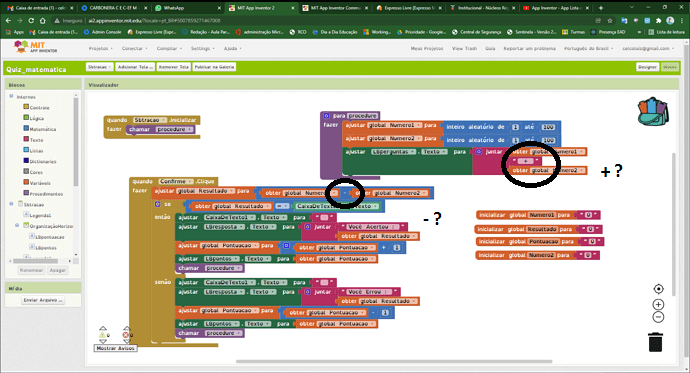It looks like this math test is asking to add two numbers but checking the result against the subtraction of those two numbers.

(sample video)

1 Like

The `abs` or `absolute` maths blocks will convert any negative number to a positive number.

1 Like

Okay, now I see the problem better.

To force random number1 > random number2,

set number3 to random(1 to 100)
set number4 to random(1 to 100)
set number1 to max(number3, number4)
set number2 to min(number3, number4)

P.S. This can be done without extra variables, if you apply a test to see if number1 < number2, then apply this trick:

P.P.S. An even easier way to force Number1 > Number2:
(editted for strict > )
set number2 to random number between 1 and 99
set number1 to random number between number2 + 1 and 100

That will force number1 > number 2.

1 Like

It would be helpful if you could temporarily change your blocks language to English, so we can take a look into your code.

Thank you. I've modified your code as per your needs, and cleaned it up a little. You can drag the images (individually) into the Blocks section, so you don't have to do everything by hand: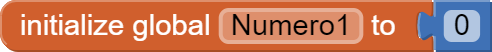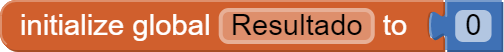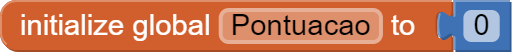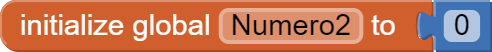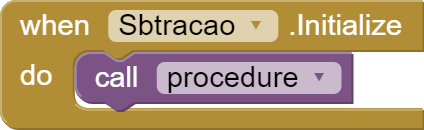you can't. You can only do this with individual block images (in this case there should be 7)